• ### ofelia lua table and a few questions

For a first understanding of ofelia 2 and lua i studied ofelia-object-help.pd and tried to "simulate" a pd array with a lua table.
Somehow its not possible to use {} inside pure data objects or messages, otherwise i would put the lua script (while its short) directly into the [ofelia define] object.
ofelia_array.pd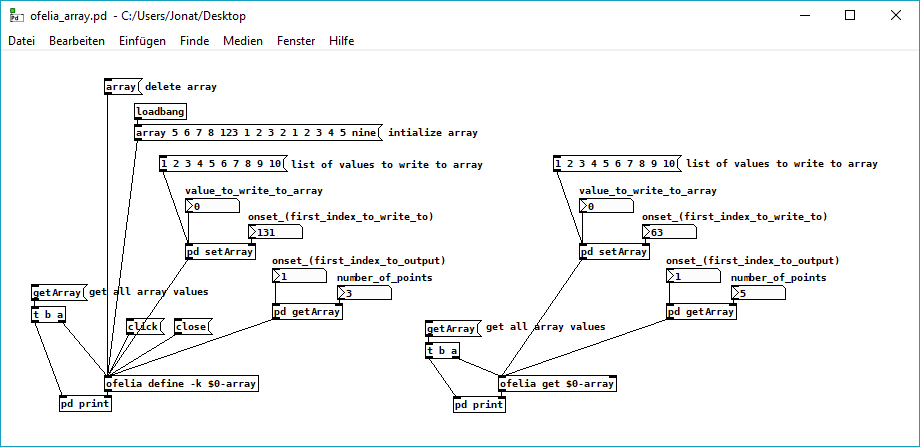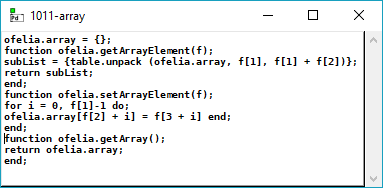@cuinjune i have a few questions regarding ofelia 2 if it doesnt matter:
Is there a way to use the [ofelia] variables outside of the [ofelia] objects (like with the @ variables and [ofSnd] / [.ofRcv] / [ofValue] objects in the old version)?
Is [ofExpr] and [ofExpr~] replaced by [ofelia define] (see [inlets_and_outlets] example in the help file)?
Do you have any plans to port the pdgui abstractions in the future? I really like them, and also would like to port the multitoggle/slider/radio abstractions as a first project to the new version. And the pdgui abstractions were a very good starting point for that.
Creating of. abstractions doesnt look that difficult, but i still have to understand it in detail.
And thanks for the great library, still need to learn a lot (lua and open frameworks)...

• Posts 18 | Views 1287
• @cuinjune i took a look into ofeliaBindings.h and i tried to make a list of the classes and methods that call internal pd methods.
i am not sure if it is correct / complete but at least it is an orientation for me.

``````class Canvas
int getIndex()
void getArgs(int *argcp, t_atom **argvp, t_canvas **canvasp)
void setArgs(int argc, t_atom *argv)
void getPosition(int **posp)
void setPosition(int xpos, int ypos)
void remove()

class Send
void sendBang()sendFloat(t_floatarg f)
void sendSymbol(t_symbol *s)
void sendPointer(t_gpointer *p)
void sendList(int argc, t_atom *argv)
void sendAnything(int argc, t_atom *argv)

class Inlet
void setFloatInlet(t_floatarg f)
void setFloatInlets(int n, t_floatarg *f)
void setSignalInlet(t_floatarg f)

class Outlet
void outletBang(int index)
void outletFloat(int index, t_floatarg f)
void outletSymbol(int index, t_symbol *s)
void outletPointer(int index, t_gpointer *p)
void outletList(int index, int argc, t_atom *argv)
void outletAnything(int index, int argc, t_atom *argv)

class Value
void set(t_floatarg f)

class Array
float getAt(int n)
float getAt(int n)
void getTable(t_word **vecp, int *sizep)
void setTable(int n, t_floatarg *f)
int getSize()
void setSize(long n)

class Clock
void delay(double delayTime)
void unset()

class Sys
double getRealTime()
void lock()
void unlock()
int tryLock()
void gui(t_symbol *s)

class PD
int getMaxString()
int getFloatSize()
t_float getMinFloat()
t_float getMaxFloat()
bool isBigOrSmall(t_floatarg f)
tuple<int, int, int> getVersion()

``````
• @Jona Yes, If you added all methods under `public:` and not `private:` then it should be the correct and complete list.

But I noticed some methods are missing. (e.g. getName() in Canvas, get() in Value..)
You need to include all methods regardless of the return type.

Thank you for making the list. I think it will be useful for other users and I need to document it inside Ofelia later.

• second try. i commented out the lines where i am not sure if they are a methods (i am quite new to programming except for pure data and a little bit of python). there are only "public:" methods in the list.

``````class Canvas
// Canvas(t_symbol *s)
// Canvas(t_symbol *s, t_floatarg f)
t_symbol *realizeDollar(t_symbol *s)
t_symbol *getName()
int getIndex()
void getArgs(int *argcp, t_atom **argvp, t_canvas **canvasp)
void setArgs(int argc, t_atom *argv)
void getPosition(int **posp)
void setPosition(int xpos, int ypos)
t_symbol *getDir()
void remove()

class Send
// Send(t_symbol *s)
void sendBang()sendFloat(t_floatarg f)
void sendSymbol(t_symbol *s)
void sendPointer(t_gpointer *p)
void sendList(int argc, t_atom *argv)
void sendAnything(int argc, t_atom *argv)

class Inlet
// Inlet(t_symbol *s)
void setFloatInlet(t_floatarg f)
void setFloatInlets(int n, t_floatarg *f)
void setSignalInlet(t_floatarg f)

class Outlet
// Outlet(t_symbol *s)
void outletBang(int index)
void outletFloat(int index, t_floatarg f)
void outletSymbol(int index, t_symbol *s)
void outletPointer(int index, t_gpointer *p)
void outletList(int index, int argc, t_atom *argv)
void outletAnything(int index, int argc, t_atom *argv)

class Value
// Value(t_symbol *s)
virtual ~Value()
t_float get()
void set(t_floatarg f)

class Array
// Array(t_symbol *s)
bool exists(t_garray **a)
float getAt(int n)
float getAt(int n)
void getTable(t_word **vecp, int *sizep)
void setTable(int n, t_floatarg *f)
int getSize()
void setSize(long n)

class Clock
// Clock(t_symbol *s)
// Clock(t_symbol *s, t_symbol *s2)
virtual ~Clock()
void delay(double delayTime)
void unset()

class Sys
double getRealTime()
void lock()
void unlock()
int tryLock()
void gui(t_symbol *s)

class Signal
int getBlockSize()
t_float getSampleRate()
int getInChannels()
int getOutChannels()
bool getDspState()

class PD
int getMaxString()
int getFloatSize()
t_float getMinFloat()
t_float getMaxFloat()
bool isBigOrSmall(t_floatarg f)
tuple<int, int, int> getVersion()
// int maxString;
// int floatSize;
// t_float minFloat;
// t_float maxFloat;
// tuple<int, int, int> version;

class Log
void post(const char *s)
void post(const char *s, int level)
void startPost(const char *s)
void postString(const char *s)
void postFloat(t_floatarg f)
void postAtom(int argc, t_atom *argv)
void endPost()
void error(const char *s)
``````
• @cuinjune it could be nice to emulate the "array set" and "array get" (with the functionality they have in pure data) in addition or instead of the tabread or tabwrite emulation if that is possible. i don´t know if that makes sense? the "array set" emulation in pdexample.pd only changes the arraysize, but doesnt set the values at the moment.

• @Jona Hi, I think I added them to pdexample patch.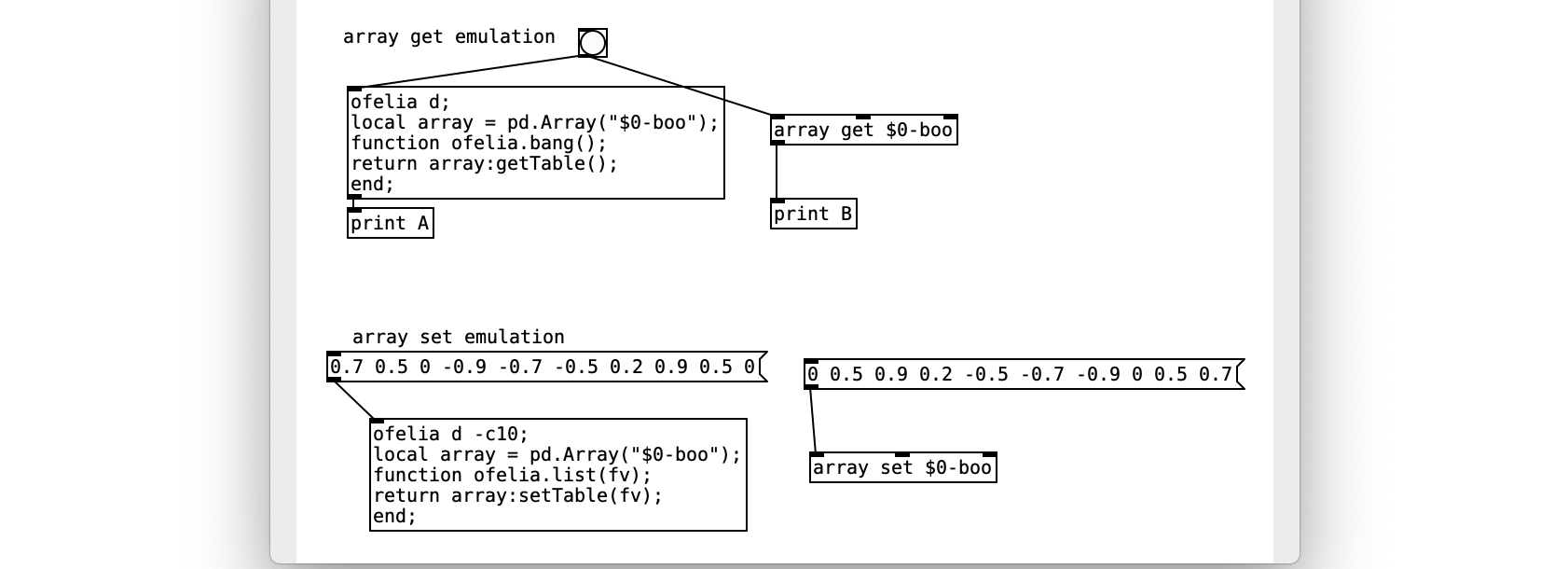Does this not work?

• @cuinjune hi. "array get emulation" works but outputs the whole array, while with the pure data "array get" you can also set "first index to output" and "number of points". same for the pure data "array set" while the number of points is defined by the length of the input list and the array isnt resized (the set values are inserted).
array set emulation doesnt work for me, it just resizes the array (win10 pd64bit).
but should define a new array and not just insert some values?
here i tried to emulate pure data "array set" with the "setAt" method:
array_set_emulation.pd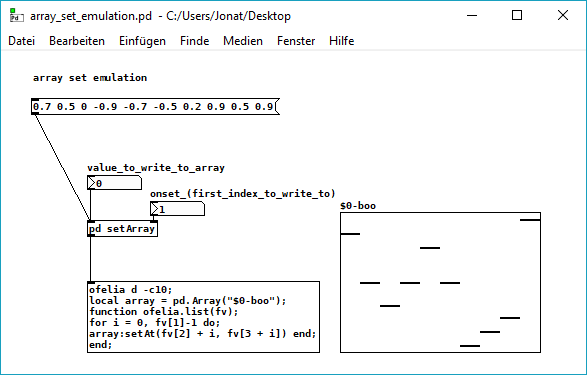but the last value of the list is always written to the last value of the array, doesnt matter which onset value is set.
i think because of the code in ofeliaBindings.h "n=size-1" which is meant for setting a single value?

``````void setAt(int n, t_floatarg f)
{
t_garray *a; int size; t_word *vec;
if (exists(&a) && getData(a, &size, &vec))
{
if (n < 0) n = 0;
else if (n >= size) n = size - 1;
vec[n].w_float = f;
garray_redraw(a);
}`````````
• @Jona Hi, I can confirm that pd.Array's setTable() function doesn't work properly on Windows.
Like you said, It seems to only change the array size.
I tested this on Windows, macOS and Linux and strangely this only happens on Windows.

I will work on fixing it as soon as possible.
Thanks!

• @Jona I edited your array set emulation patch and it seems to work.
array_set_emulation.pd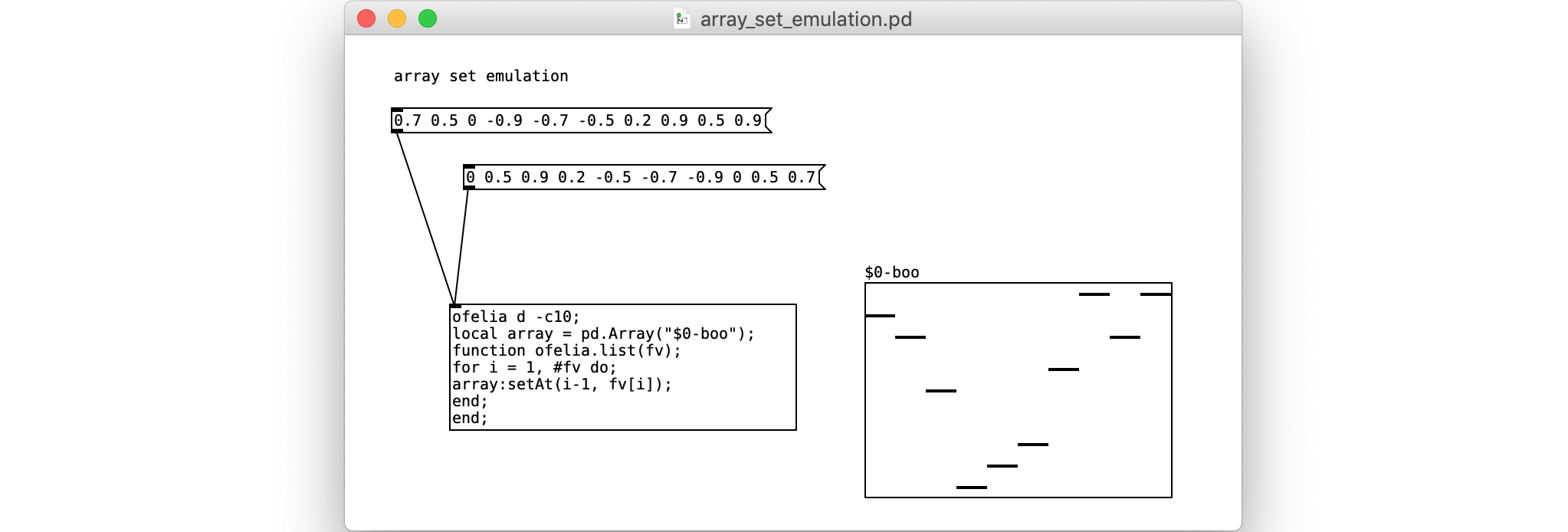• hi @cuinjune thanks for the edited array_set_emulation patch, it works nowi will post the pd.Array's setTable() issue on your github page soon.
edit: just realized that your array set emulation only seems to work if the onset value is zero too, but perhaps the onset option isnt needed anyways (or there are other ways to achieve that).

• @Jona Hi, I added the onset option.array_set_emulation.pd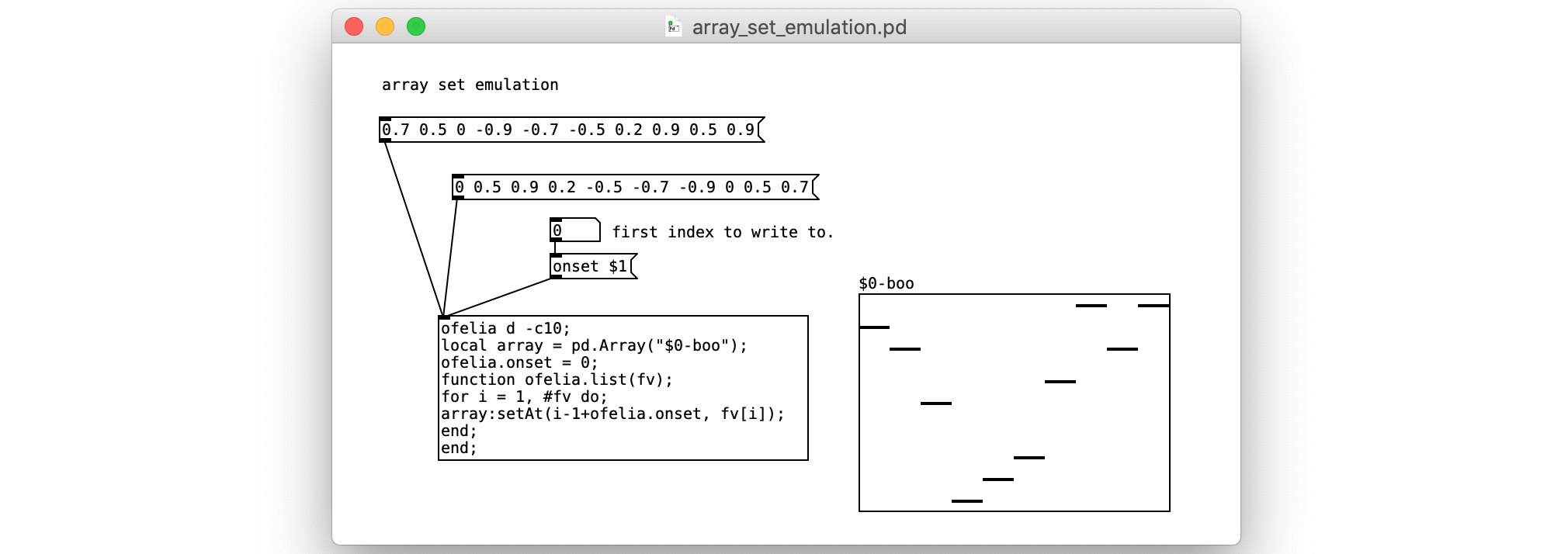• @cuinjune thankssomehow i still get the same "bug" as i got in my attempt. the last value of the input list is always set to the last value of the array independently from the onset (if there are more values in the input list than are in the table left after the onset)... but perhaps its again a windows specific problem?

• @Jona I'm sorry that happens when calling array:setAt() using the index larger than the array size in which case it internally clips the index to the "arraysize -1" (or last index).

This version will fix the issue. Let me know if it didn't.
array_set_emulation2.pd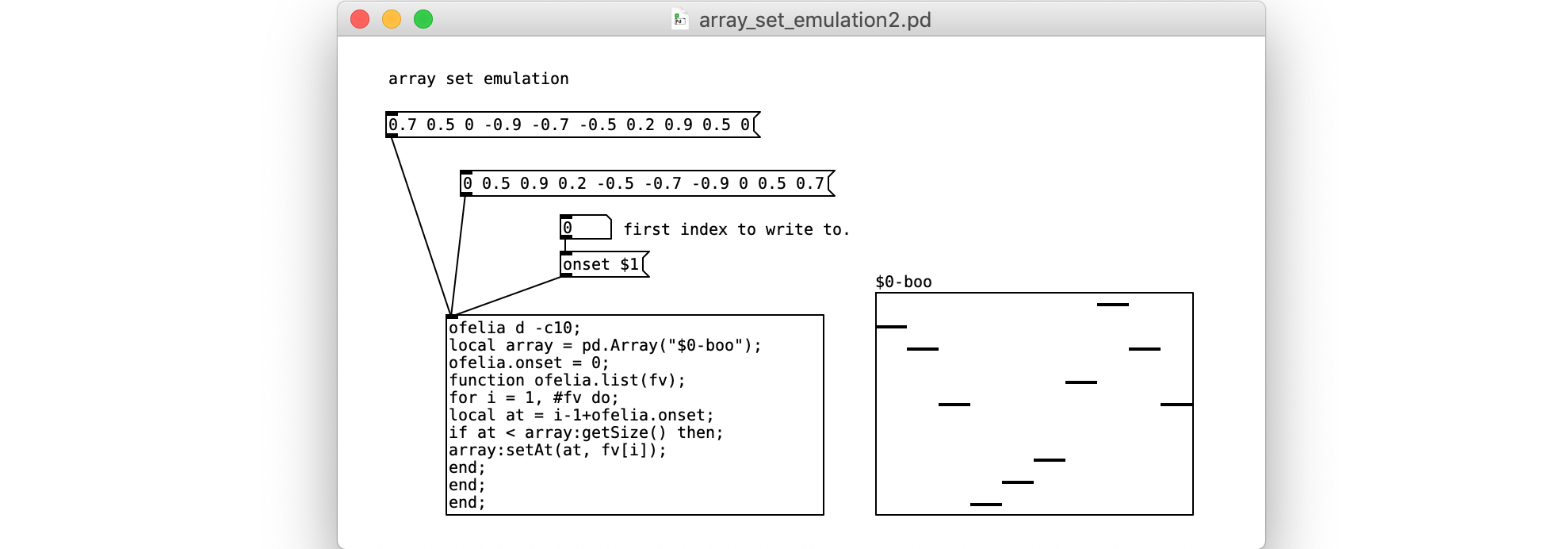• yes, thats it. thankssorry if i didnt explain my (theoretical) problem precise enough.

Posts 18 | Views 1287
Internal error.

Oops! Looks like something went wrong!